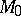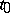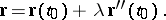# Principal normal

A normal to a curvepassing through a pointofand lying in the osculating plane toat. Ifis the parametric equation of the curve and the valuecorresponds to, then the equation of the principal normal in vector form is: